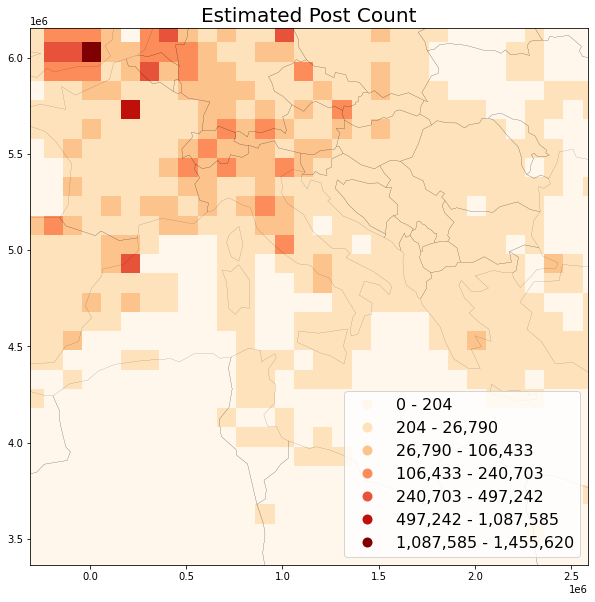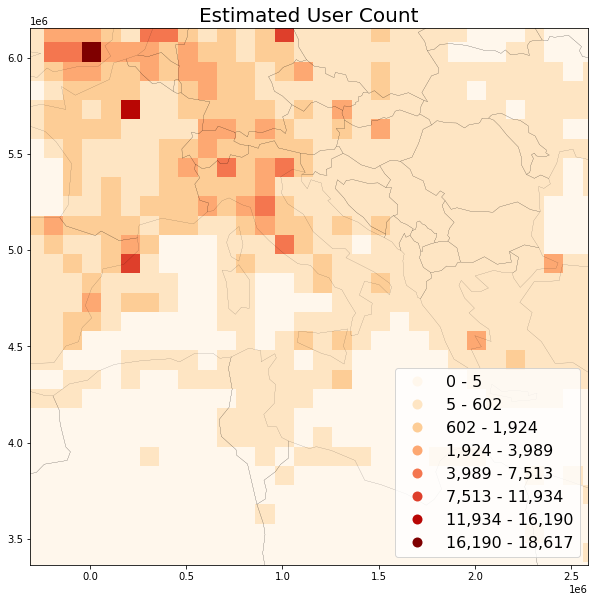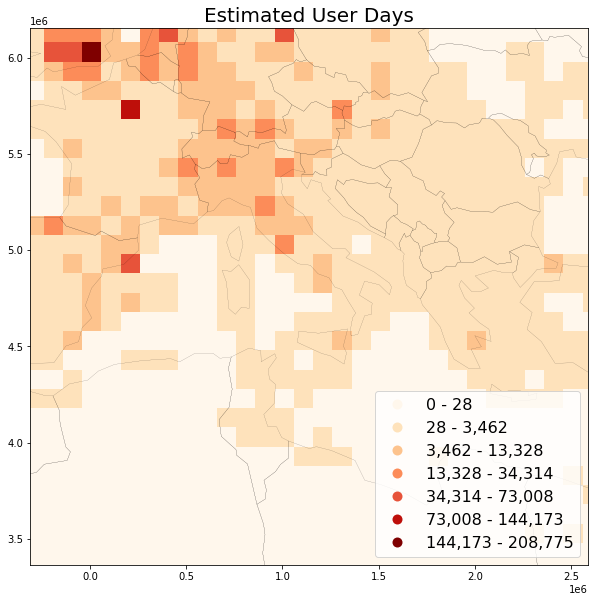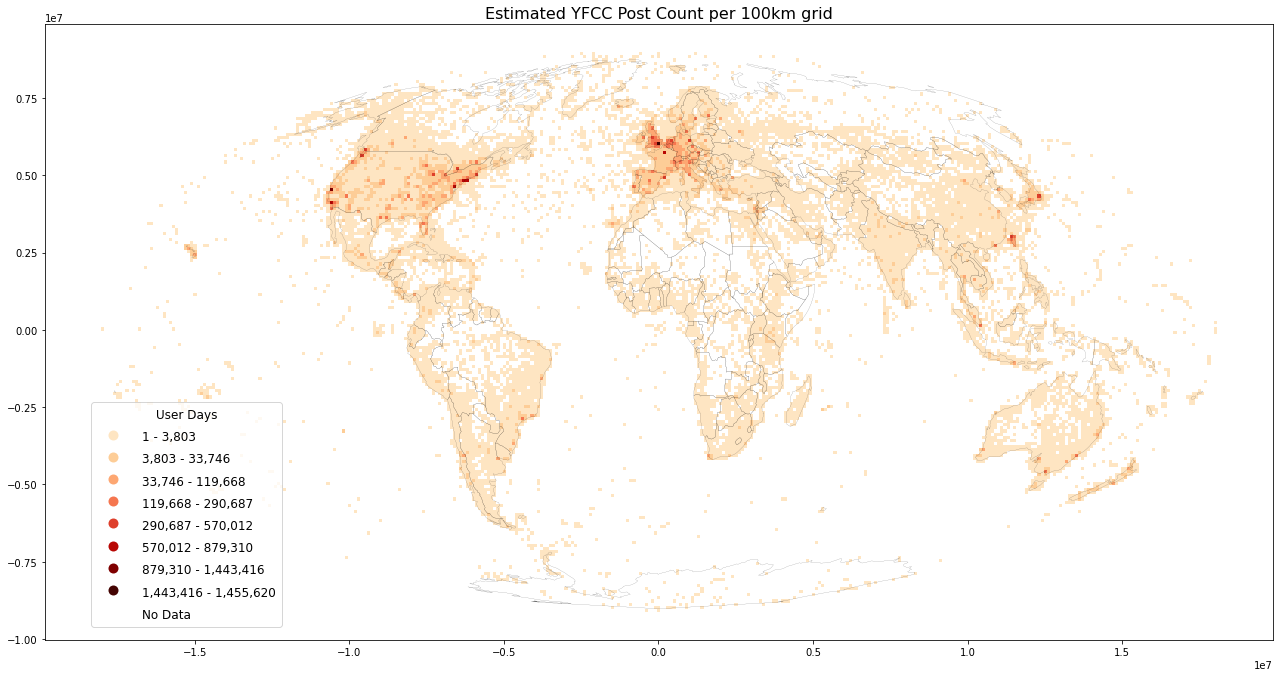# Introduction¶

Based on data from YFCC100m dataset, this Notebook explores a privacy-aware processing example for visualizing frequentation patterns in a 100x100km Grid (worldwide).

This is the third notebook in a series of four notebooks:

This notebook includes code parts and examples that have nothing to do with HyperLogLog. Our goal was to illustrate a complete typical visualization pipeline, from reading data to processing to visualization. There're additional steps included such as archiving intermediate results or creating an alternative interactive visualization. At the various parts, we discuss advantages and disadvantages of the privacy-aware data structure compared to working with raw data.

In this Notebook, we describe a complete visualization pipeline, exploring worldwide frequentation patterns from YFCC dataset based on a 100x100km grid. In addition to the steps listed in the raw notebook, this notebooks describes, among other aspects:

• get data from LBSN hll db (PostgreSQL select)
• store hll data to CSV, load from CSV
• incremental union of hll sets
• estimated cardinality for metrics postcount, usercount and userdays
• measure timing of different steps, to compare processing time with raw-dataset approach
• load and store intermediate results from and to *.pickle and *.CSV
• create benchmark data to be published

System requirements

The Notebook is configured to run on a computer with 8 GB of Memory (minimum).

If more is available, you may increase the chunk_size parameter (Default is 5000000 records per chunk) to improve speed.

Use Shift+Enter to walk through the Notebook

# Preparations¶

Dependencies are already defined in 02_yfcc_gridagg_raw.ipynb. We're loading the jupytext python converted version of this notebook to the main namespace, which will make all methods and parameters available here.

In :
import sys
from pathlib import Path
module_path = str(Path.cwd().parents / "py")
if module_path not in sys.path:
sys.path.append(module_path)

from _02_yfcc_gridagg_raw import *
from modules import tools, preparations


## Parameters¶

Parameters from the first notebook are available through import. They can be overridden here.

GRID_SIZE_METERS = 100000 # the size of grid cells in meters # (spatial accuracy of worldwide measurement) CHUNK_SIZE = 5000000 # process x number of hll records per chunk. # Increasing this number will consume more memory, # but reduce processing time because less SQL queries # are needed.

Activate autoreload of changed python files:

In :
%load_ext autoreload


Load memory profiler extension

In :
%load_ext memory_profiler


Plot used package versions for future use:

•••
package xarray Shapely pyproj pandas numpy matplotlib mapclassify ipython holoviews geoviews geopandas Fiona Cartopy bokeh
version 0.16.1 1.7.1 2.6.1.post1 1.1.2 1.19.1 3.3.2 2.3.0 7.18.1 1.13.4 1.8.1 0.8.1 1.8.17 0.18.0 2.2.1

### Connect to database¶

The password is automatically loaded from .env file specified in container setup hlldb.

The docker stack contains a full backup of the YFCC database converted to the privacy-aware datastructure. In this Notebook, we're only working with a small part of the data from the table spatial.latlng.

Define credentials as environment variables

In :
db_user = "postgres"
# set connection variables
db_host = "hlldb"
db_port = "5432"
db_name = "hlldb"


Connect to empty Postgres database running HLL Extension. Note that only read privileges are needed.

In :
db_connection = psycopg2.connect(
host=db_host,
port=db_port,
dbname=db_name,
user=db_user,
)


Test connection:

In :
db_query = """
SELECT 1;
"""
# create pandas DataFrame from database data
df = pd.read_sql_query(db_query, db_connection)

?column?
0 1

For simplicity, the db connection parameters and query are stored in a class:

In :
db_conn = tools.DbConn(db_connection)
db_conn.query("SELECT 1;")

Out:
?column?
0 1

## Get data from db and write to CSV¶

To compare processing speed with the raw notebook, we're also going to save hll data to CSV first. The following records are available from table spatial.latlng:

• distinct latitude and longitude coordinates (clear text), this is the "base" we're working on
• post_hll - approximate post guids stored as hll set
• user_hll - approximate user guids stored as hll set
• date_hll - approximate user days stored as hll set
In :
def get_yfccposts_fromdb(
chunk_size: int = CHUNK_SIZE) -> List[pd.DataFrame]:
"""Returns spatial.latlng data from db, excluding Null Island"""
sql = f"""
SELECT  latitude,
longitude,
post_hll,
user_hll,
date_hll
FROM spatial.latlng t1
WHERE
NOT ((latitude = 0) AND (longitude = 0));
"""
# execute query, enable chunked return
return pd.read_sql(sql, con=db_connection, chunksize=chunk_size)


Execute Query:

In :
%%time
filename = "yfcc_latlng.csv"
usecols = ["latitude", "longitude", "post_hll", "user_hll", "date_hll"]
if Path(OUTPUT / "csv" / filename).exists():
print(f"CSV already exists, skipping load from db.. (to reload, delete file)")
else:
write_chunkeddf_tocsv(
chunked_df=get_yfccposts_fromdb(),
filename=filename,
usecols=usecols)

'Stored 451949 posts to CSV..'
CPU times: user 9.47 s, sys: 1.04 s, total: 10.5 s
Wall time: 12.1 s


HLL file size:

In :
hll_size_mb = Path(OUTPUT / "csv" / "yfcc_latlng.csv").stat().st_size / (1024*1024)
print(f"Size: {hll_size_mb:.2f} MB")

Size: 134.37 MB


## Create Grid¶

In :
grid, rows, cols = create_grid_df(
report=True, return_rows_cols=True)

361
181

In :
grid = grid_to_gdf(grid)


Add columns for aggregation

In :
METRICS = ["postcount_est", "usercount_est", "userdays_est"]

In :
reset_metrics(grid, METRICS)
display(grid)

geometry
xbin ybin
-18040096 8979952 POLYGON ((-18040096.000 8979952.000, -17940096...
8879952 POLYGON ((-18040096.000 8879952.000, -17940096...
8779952 POLYGON ((-18040096.000 8779952.000, -17940096...
8679952 POLYGON ((-18040096.000 8679952.000, -17940096...
8579952 POLYGON ((-18040096.000 8579952.000, -17940096...
... ... ...
17959904 -8620048 POLYGON ((17959904.000 -8620048.000, 18059904....
-8720048 POLYGON ((17959904.000 -8720048.000, 18059904....
-8820048 POLYGON ((17959904.000 -8820048.000, 18059904....
-8920048 POLYGON ((17959904.000 -8920048.000, 18059904....
-9020048 POLYGON ((17959904.000 -9020048.000, 18059904....

65341 rows × 1 columns

Read World geometries data

In :
%%time
world = gp.read_file(gp.datasets.get_path('naturalearth_lowres'), crs=CRS_WGS)
world = world.to_crs(CRS_PROJ)

CPU times: user 315 ms, sys: 27.2 ms, total: 342 ms
Wall time: 339 ms


## Prepare functions¶

Now that it has been visually verified that the algorithm works. We'll use some of the functions defined in the previous notebook.

## Test with LBSN data¶

We're going to test the binning of coordinates on a part of the YFCC geotagged images.

Create 2 bins for each axis of existing Mollweide rows/cols grid:

In :
ybins = np.array(rows)
xbins = np.array(cols)


Prepare lat/lng tuple of lower left corner and upper right corner to crop sample map:

In :
# Part of Italy and Sicily
bbox_italy = (
7.8662109375, 36.24427318493909,
19.31396484375, 43.29320031385282)
bbox = bbox_italy


Calculate bounding box with 1000 km buffer. For that, project the bounding Box to Mollweide, apply the buffer, and project back to WGS1984:

In :
# convert to Mollweide
minx, miny = PROJ_TRANSFORMER.transform(
bbox_italy, bbox_italy)
maxx, maxy = PROJ_TRANSFORMER.transform(
bbox_italy, bbox_italy)
buf = 1000000
# apply buffer and convetr back to WGS1984
min_buf = PROJ_TRANSFORMER_BACK.transform(minx-buf, miny-buf)
max_buf = PROJ_TRANSFORMER_BACK.transform(maxx+buf, maxy+buf)
bbox_italy_buf = [min_buf, min_buf, max_buf, max_buf]


Select columns and types for improving speed

In :
usecols = ['latitude', 'longitude', 'post_hll']
dtypes = {'latitude': float, 'longitude': float}
reset_metrics(grid, METRICS)


In :
%%time
OUTPUT / "csv" / "yfcc_latlng.csv", usecols=usecols, dtype=dtypes, encoding='utf-8')
print(len(df))

451949
CPU times: user 2.77 s, sys: 150 ms, total: 2.92 s
Wall time: 2.92 s


Execute and count number of posts in the bounding box:

In :
%%time
filter_df_bbox(df=df, bbox=bbox_italy_buf)
print(f"There're {len(df):,.0f} YFCC distinct lat-lng coordinates located within the bounding box.")

There're 92,775 YFCC distinct lat-lng coordinates located within the bounding box.
CPU times: user 87.8 ms, sys: 386 µs, total: 88.2 ms
Wall time: 84.8 ms

Out:
latitude longitude post_hll
0 27.663574 28.410645 \x138b40ba01
1 27.663574 30.915527 \x138b4001e4040204e20a8114231b012bc137e33d6246...
2 27.663574 30.959473 \x138b4025a144c2
3 27.663574 33.596191 \x138b40068535c1452157a6af63fb03
4 27.663574 33.728027 \x138b40f3e1

### Project coordinates to Mollweide¶

In :
%%time
proj_df(df)
print(f'Projected {len(df.values)} coordinates')

Projected 92775 coordinates
CPU times: user 129 ms, sys: 4.04 ms, total: 133 ms
Wall time: 131 ms

Out:
post_hll x y
0 \x138b40ba01 2.641304e+06 3.369176e+06
1 \x138b4001e4040204e20a8114231b012bc137e33d6246... 2.874180e+06 3.369176e+06
2 \x138b4025a144c2 2.878265e+06 3.369176e+06
3 \x138b40068535c1452157a6af63fb03 3.123398e+06 3.369176e+06
4 \x138b40f3e1 3.135655e+06 3.369176e+06

### Perform the bin assignment¶

In :
%%time
xbins_match, ybins_match = get_best_bins(
search_values_x=df['x'].to_numpy(),
search_values_y=df['y'].to_numpy(),
xbins=xbins, ybins=ybins)

CPU times: user 6.34 ms, sys: 3.81 ms, total: 10.2 ms
Wall time: 8.37 ms

In :
len(xbins_match)

Out:
92775
In :
xbins_match[:10]

Out:
array([2559904, 2859904, 2859904, 3059904, 3059904, 3059904, 3159904,
2559904, 2659904, 3159904])
In :
ybins_match[:10]

Out:
array([3379952, 3379952, 3379952, 3379952, 3379952, 3379952, 3379952,
3379952, 3379952, 3379952])

## A: Estimated Post Count per grid¶

Attach target bins to original dataframe. The : means: modify all values in-place

In :
df.loc[:, 'xbins_match'] = xbins_match
df.loc[:, 'ybins_match'] = ybins_match
# set new index column
df.set_index(['xbins_match', 'ybins_match'], inplace=True)
# drop x and y columns not needed anymore
df.drop(columns=['x', 'y'], inplace=True)

In :
df.head()

Out:
post_hll
xbins_match ybins_match
2559904 3379952 \x138b40ba01
2859904 3379952 \x138b4001e4040204e20a8114231b012bc137e33d6246...
3379952 \x138b4025a144c2
3059904 3379952 \x138b40068535c1452157a6af63fb03
3379952 \x138b40f3e1

The next step is to union hll sets and (optionally) return the cardinality (the number of distinct elements). This can be done by connecting to a postgres database with HLL extension installed. There's a python package available for HLL calculations, but it is in a very early stage of development. For simplicity, we're using our hlldb here, but it is equally possible to connect to an empty Postgres DB running Citus HLL such as pg-hll-empty docker container.

In :
def union_hll(
hll_series: pd.Series, cardinality: bool = True) -> pd.Series:
"""HLL Union and (optional) cardinality estimation from series of hll sets
based on group by composite index.

Args:
hll_series: Indexed series (bins) of hll sets.
cardinality: If True, returns cardinality (counts). Otherwise,
the unioned hll set will be returned.

The method will combine all groups of hll sets first,
in a single SQL command. Union of hll hll-sets belonging
to the same group (bin) and (optionally) returning the cardinality
(the estimated count) per group will be done in postgres.

By utilizing Postgres´ GROUP BY (instead of, e.g. doing
the group with numpy), it is possible to reduce the number
of SQL calls to a single run, which saves overhead
(establishing the db connection, initializing the SQL query
etc.). Also note that ascending integers are used for groups,
instead of their full original bin-ids, which also reduces
transfer time.

cardinality = True should be used when calculating counts in
a single pass.

cardinality = False should be used when incrementally union
of hll sets is required, e.g. due to size of input data.
In the last run, set to cardinality = True.
"""
# group all hll-sets per index (bin-id)
series_grouped = hll_series.groupby(
hll_series.index).apply(list)
# From grouped hll-sets,
# construct a single SQL Value list;
# if the following nested list comprehension
# doesn't make sense to you, have a look at
# spapas.github.io/2016/04/27/python-nested-list-comprehensions/
# with a decription on how to 'unnest'
# nested list comprehensions to regular for-loops
hll_values_list = ",".join(
[f"({ix}::int,'{hll_item}'::hll)"
for ix, hll_items
in enumerate(series_grouped.values.tolist())
for hll_item in hll_items])
# Compilation of SQL query,
# depending on whether to return the cardinality
# of unioned hll or the unioned hll
return_col = "hll_union"
hll_calc_pre = ""
hll_calc_tail = "AS hll_union"
if cardinality:
# add sql syntax for cardinality
# estimation
# (get count distinct from hll)
return_col = "hll_cardinality"
hll_calc_pre = "hll_cardinality("
hll_calc_tail = ")::int"
db_query = f"""
SELECT sq.{return_col} FROM (
SELECT s.group_ix,
{hll_calc_pre}
hll_union_agg(s.hll_set)
{hll_calc_tail}
FROM (
VALUES {hll_values_list}
) s(group_ix, hll_set)
GROUP BY group_ix
ORDER BY group_ix ASC) sq
"""
df = db_conn.query(db_query)
# to merge values back to grouped dataframe,
# first reset index to ascending integers
# matching those of the returned df;
# this will turn series_grouped into a DataFrame;
# the previous index will still exist in column 'index'
df_grouped = series_grouped.reset_index()
# drop hll sets not needed anymore
df_grouped.drop(columns=[hll_series.name], inplace=True)
# append hll_cardinality counts
# using matching ascending integer indexes
df_grouped.loc[df.index, return_col] = df[return_col]
# set index back to original bin-ids
df_grouped.set_index("index", inplace=True)
# split tuple index to produce
# the multiindex of the original dataframe
# with xbin and ybin column names
df_grouped.index = pd.MultiIndex.from_tuples(
df_grouped.index, names=['xbin', 'ybin'])
# return column as indexed pd.Series
return df_grouped[return_col]


Optionally, split dataframe into chunks, so we're not the exceeding memory limit (e.g. use if memory < 16GB). A chunk size of 1 Million records is suitable for a computer with about 8 GB of memory and optional sparse HLL mode enabled. If sparse mode is disabled, decrease chunk_size accordingly, to compensate for increased space.

In :
%%time
chunked_df = [
df[i:i+CHUNK_SIZE] for i in range(0, df.shape, CHUNK_SIZE)]

CPU times: user 765 µs, sys: 0 ns, total: 765 µs
Wall time: 783 µs

In :
chunked_df.head()

Out:
post_hll
xbins_match ybins_match
2559904 3379952 \x138b40ba01
2859904 3379952 \x138b4001e4040204e20a8114231b012bc137e33d6246...
3379952 \x138b4025a144c2
3059904 3379952 \x138b40068535c1452157a6af63fb03
3379952 \x138b40f3e1

To test, process the first chunk:

In :
%%time
cardinality_series = union_hll(chunked_df["post_hll"])

CPU times: user 906 ms, sys: 26.7 ms, total: 932 ms
Wall time: 3.72 s

In :
cardinality_series.head()

Out:
xbin     ybin
-340096  3879952       3
4179952       1
4279952     971
4379952       1
4479952    6449
Name: hll_cardinality, dtype: int64

Remove possibly existing result column in grid from previous run:

In :
reset_metrics(grid, ["postcount_est"], setzero=True)


Append Series with calculated counts to grid (as new column) based on index match:

In :
grid.loc[cardinality_series.index, 'postcount_est'] = cardinality_series

In :
grid[grid["postcount_est"] > 0].head()

Out:
geometry postcount_est
xbin ybin
-340096 6179952 POLYGON ((-340096.000 6179952.000, -240096.000... 772
6079952 POLYGON ((-340096.000 6079952.000, -240096.000... 12926
5979952 POLYGON ((-340096.000 5979952.000, -240096.000... 5181
5879952 POLYGON ((-340096.000 5879952.000, -240096.000... 1
5779952 POLYGON ((-340096.000 5779952.000, -240096.000... 1531

Process all chunks:

The caveat here is to incrementally union hll sets until all records have been processed. On the last loop, instruct the hll worker to return the cardinality instead of the unioned hll set.

First, define method to join cardinality to grid

In :
# reference metric names and column names
COLUMN_METRIC_REF = {
"postcount_est":"post_hll",
"usercount_est":"user_hll",
"userdays_est":"date_hll"}

def join_df_grid(
df: pd.DataFrame, grid: gp.GeoDataFrame,
metric: str = "postcount_est",
cardinality: bool = True,
column_metric_ref: Dict[str,str] = COLUMN_METRIC_REF):
"""Union HLL Sets and estimate postcount per
grid bin from lat/lng coordinates

Args:
df: A pandas dataframe with latitude and
longitude columns in WGS1984
grid: A geopandas geodataframe with indexes
x and y (projected coordinates) and grid polys
metric: target column for estimate aggregate.
Default: postcount_est.
cardinality: will compute cardinality of unioned
hll sets. Otherwise, unioned hll sets will be
returned for incremental updates.
"""
# optionally, bin assigment of projected coordinates,
# make sure to not bin twice:
# x/y columns are removed after binning
if 'x' in df.columns:
bin_coordinates(df, xbins, ybins)
# set index column
df.set_index(
['xbins_match', 'ybins_match'], inplace=True)
# union hll sets and
# optional estimate count distincts (cardinality)
column = column_metric_ref.get(metric)
# get series with grouped hll sets
hll_series = df[column]
# union of hll sets:
# to allow incremental union of already merged data
# and new data, concatenate series from grid and new df
# only if column with previous hll sets already exists
if metric in grid.columns:
# remove nan values from grid and
# rename series to match names
hll_series = pd.concat(
[hll_series, grid[metric].dropna()]
).rename(column)
cardinality_series = union_hll(
hll_series, cardinality=cardinality)
# add unioned hll sets/computed cardinality to grid
grid.loc[
cardinality_series.index, metric] = cardinality_series
if cardinality:
# set all remaining grid cells
# with no data to zero and
# downcast column type from float to int
grid[metric] = grid[metric].fillna(0).astype(int)


Define method to process chunks:

In :
def join_chunkeddf_grid(
chunked_df: List[pd.DataFrame], grid: gp.GeoDataFrame,
metric: str = "postcount_est", chunk_size: int = CHUNK_SIZE,
benchmark_data: Optional[bool] = None,
column_metric_ref: Dict[str,str] = COLUMN_METRIC_REF):
"""Incremental union of HLL Sets and estimate postcount per
grid bin from chunked list of dataframe records. Results will
be stored in grid.

Args:
chunked_df: A list of (chunked) dataframes with latitude and
longitude columns in WGS1984
grid: A geopandas geodataframe with indexes
x and y (projected coordinates) and grid polys
metric: target column for estimate aggregate.
Default: postcount_est.
benchmark_data: If True, will not remove HLL sketches after
final cardinality estimation.
column_metric_ref: Dictionary containing references of
metrics to df columns.
"""
reset_metrics(grid, [metric])
for ix, chunk_df in enumerate(chunked_df):
# compute cardinality only on last iteration
cardinality = False
if ix == len(chunked_df)-1:
cardinality = True
column = column_metric_ref.get(metric)
# get series with grouped hll sets
hll_series = chunk_df[column]
if metric in grid.columns:
# merge existing hll sets with new ones
# into one series (with duplicate indexes);
# remove nan values from grid and
# rename series to match names
hll_series = pd.concat(
[hll_series, grid[metric].dropna()]
).rename(column)
cardinality_series = union_hll(
hll_series, cardinality=cardinality)
if benchmark_data and (ix == len(chunked_df)-1):
# only if final hll sketches need to
# be kept for benchmarking:
# do another union, without cardinality
# estimation, and store results
# in column "metric"_hll
hll_sketch_series = union_hll(
hll_series, cardinality=False)
grid.loc[
hll_sketch_series.index,
f'{metric.replace("_est","_hll")}'] = hll_sketch_series
# add unioned hll sets/computed cardinality to grid
grid.loc[
cardinality_series.index, metric] = cardinality_series
if cardinality:
# set all remaining grid cells
# with no data to zero and
# downcast column type from float to int
grid[metric] = grid[metric].fillna(0).astype(int)
clear_output(wait=True)
print(f'Mapped ~{(ix+1)*chunk_size} coordinates to bins')

In :
join_chunkeddf_grid(chunked_df, grid, chunk_size=CHUNK_SIZE)

Mapped ~5000000 coordinates to bins


All distinct counts are now attached to the bins of the grid:

In :
grid[grid["postcount_est"]>10].head()

Out:
geometry postcount_est
xbin ybin
-340096 6179952 POLYGON ((-340096.000 6179952.000, -240096.000... 772
6079952 POLYGON ((-340096.000 6079952.000, -240096.000... 12926
5979952 POLYGON ((-340096.000 5979952.000, -240096.000... 5181
5779952 POLYGON ((-340096.000 5779952.000, -240096.000... 1531
5679952 POLYGON ((-340096.000 5679952.000, -240096.000... 6965

### Preview post count map¶

Use headtail_breaks classification scheme because it is specifically suited to map long tailed data, see Jiang 2013

• Jiang, B. (August 01, 2013). Head/Tail Breaks: A New Classification Scheme for Data with a Heavy-Tailed Distribution. The Professional Geographer, 65, 3, 482-494.
In :
# global legend font size setting
plt.rc('legend', **{'fontsize': 16})

In :
save_plot(
grid=grid, title='Estimated Post Count',
column='postcount_est', save_fig='postcount_sample_est.png',
bbox=bbox_italy, world=world)## B: Estimated User Count per grid¶

When using HLL, aggregation of user_guids or user_days takes the same amount of time (unlike when working with original data, where memory consumption increases significantly). We'll only need to update the columns that are loaded from the database:

In :
usecols = ['latitude', 'longitude', 'user_hll']


Adjust method for stream-reading from CSV in chunks:

In :
iter_csv = pd.read_csv(
OUTPUT / "csv" / "yfcc_latlng.csv", usecols=usecols, iterator=True,
dtype=dtypes, encoding='utf-8', chunksize=CHUNK_SIZE)

In :
%%time
# filter
chunked_df = [
filter_df_bbox(
df=chunk_df, bbox=bbox_italy_buf, inplace=False)
for chunk_df in iter_csv]

# project
projected_cnt = 0
for chunk_df in chunked_df:
projected_cnt += len(chunk_df)
proj_report(
chunk_df, projected_cnt, inplace=True)


Projected 92,775 coordinates
CPU times: user 2.02 s, sys: 189 ms, total: 2.21 s
Wall time: 2.19 s

Out:
user_hll x y
0 \x138b4054e2 2.641304e+06 3.369176e+06
1 \x138b40200439019663c582 2.874180e+06 3.369176e+06
2 \x138b40c582 2.878265e+06 3.369176e+06
3 \x138b40cbe1 3.123398e+06 3.369176e+06
4 \x138b4027c3 3.135655e+06 3.369176e+06

### Perform the bin assignment and estimate distinct users¶

In :
%%time
bin_chunked_coordinates(chunked_df)

Binned 92,775 coordinates..
CPU times: user 32.2 ms, sys: 3.76 ms, total: 36 ms
Wall time: 33.5 ms

Out:
user_hll
xbins_match ybins_match
2559904 3379952 \x138b4054e2
2859904 3379952 \x138b40200439019663c582
3379952 \x138b40c582
3059904 3379952 \x138b40cbe1
3379952 \x138b4027c3

Union HLL Sets per grid-id and calculate cardinality (estimated distinct user count):

In :
join_chunkeddf_grid(
chunked_df=chunked_df, grid=grid, metric="usercount_est")

Mapped ~5000000 coordinates to bins

In :
grid[grid["usercount_est"]> 0].head()

Out:
geometry postcount_est usercount_est
xbin ybin
-340096 6179952 POLYGON ((-340096.000 6179952.000, -240096.000... 772 65
6079952 POLYGON ((-340096.000 6079952.000, -240096.000... 12926 521
5979952 POLYGON ((-340096.000 5979952.000, -240096.000... 5181 232
5879952 POLYGON ((-340096.000 5879952.000, -240096.000... 1 1
5779952 POLYGON ((-340096.000 5779952.000, -240096.000... 1531 148

Look at this. There're many polygons were thounsands of photos have been created by only few users. Lets see how this affects our test map..

### Preview user count map¶

In :
save_plot(
grid=grid, title='Estimated User Count',
column='usercount_est', save_fig='usercount_sample_est.png',
bbox=bbox_italy, world=world)## C: Estimated User Days¶

Usually, due to the Count Distinct Problem increasing computation times will apply for more complex distinct queries. This is not the case when using HLL. Any count distinct (postcount, usercount etc.) requires the same amount of time. A useful metric introduced by Wood et al. (2013) is User Days, which lies inbetween Post Count and User Count because Users may be counted more than once if they visited the location on consecutive days. User Days particularly allows capturing the difference between local and tourist behaviour patterns. The rationale here is that locals visit few places very often. In contrast, tourists visit many places only once.

The sequence of commands for userdays is exactly the same as for postcount and usercount above.

In :
usecols = ['latitude', 'longitude', 'date_hll']

In :
def read_project_chunked(filename: str,
usecols: List[str], chunk_size: int = CHUNK_SIZE,
bbox: Tuple[float, float, float, float] = None,
output: Path = OUTPUT,
dtypes = None) -> List[pd.DataFrame]:
"""Read data from csv, optionally clip to bbox and projet"""
if dtypes is None:
dtypes = {'latitude': float, 'longitude': float}
output / "csv" / filename, usecols=usecols, iterator=True,
dtype=dtypes, encoding='utf-8', chunksize=chunk_size)
if bbox:
chunked_df = [filter_df_bbox(
df=chunk_df, bbox=bbox, inplace=False)
for chunk_df in iter_csv]
else:
chunked_df = [chunk_df for chunk_df in iter_csv]
# project
projected_cnt = 0
for chunk_df in chunked_df:
projected_cnt += len(chunk_df)
proj_report(
chunk_df, projected_cnt, inplace=True)
return chunked_df


Run:

In :
%%time
filename="yfcc_latlng.csv",
usecols=usecols,
bbox=bbox_italy_buf)

Projected 92,775 coordinates
CPU times: user 2.27 s, sys: 168 ms, total: 2.43 s
Wall time: 2.43 s

Out:
date_hll x y
0 \x138b40fbe2 2.641304e+06 3.369176e+06
1 \x138b4021613a629864ee41f2a1 2.874180e+06 3.369176e+06
2 \x138b40f2a1 2.878265e+06 3.369176e+06
3 \x138b4067847141b903c5e3 3.123398e+06 3.369176e+06
4 \x138b40a2e1 3.135655e+06 3.369176e+06
In :
%%time
bin_chunked_coordinates(chunked_df)

Binned 92,775 coordinates..
CPU times: user 48.1 ms, sys: 3.89 ms, total: 52 ms
Wall time: 47.7 ms

In :
join_chunkeddf_grid(
chunked_df=chunked_df, grid=grid, metric="userdays_est")

Mapped ~5000000 coordinates to bins

In :
chunked_df.head()

Out:
date_hll
xbins_match ybins_match
2559904 3379952 \x138b40fbe2
2859904 3379952 \x138b4021613a629864ee41f2a1
3379952 \x138b40f2a1
3059904 3379952 \x138b4067847141b903c5e3
3379952 \x138b40a2e1
In :
grid[grid["userdays_est"]> 0].head()

Out:
geometry postcount_est usercount_est userdays_est
xbin ybin
-340096 6179952 POLYGON ((-340096.000 6179952.000, -240096.000... 772 65 164
6079952 POLYGON ((-340096.000 6079952.000, -240096.000... 12926 521 2545
5979952 POLYGON ((-340096.000 5979952.000, -240096.000... 5181 232 778
5879952 POLYGON ((-340096.000 5879952.000, -240096.000... 1 1 1
5779952 POLYGON ((-340096.000 5779952.000, -240096.000... 1531 148 341
In :
save_plot(
grid=grid, title='Estimated User Days',
column='userdays_est', save_fig='userdays_sample_est.png',
bbox=bbox_italy, world=world)There're other approaches for further reducing noise. For example, to reduce the impact of automatic capturing devices (such as webcams uploading x pictures per day), a possibility is to count distinct userlocations. For userlocations metric, a user would be counted multiple times per grid bin only for pictures with different lat/lng. Or the number of distinct userlocationdays (etc.). These metrics easy to implement using hll, but would be quite difficult to compute using raw data.

# Prepare methods¶

Lets summarize the above code in a few methods:

Plotting preparation

The method below utilizes many of the methods defined for raw data processing.

In :
def load_plot(
grid: gp.GeoDataFrame, title: str, inverse: bool = None,
metric: str = "postcount_est", store_fig: str = None, store_pickle: str = None,
chunk_size: int = CHUNK_SIZE, benchmark_data: Optional[bool] = None,
column_metric_ref: Dict[str,str] = COLUMN_METRIC_REF):
"""Load data, bin coordinates, estimate distinct counts (cardinality) and plot map

Args:
data: Path to read input CSV
grid: A geopandas geodataframe with indexes x and y
(projected coordinates) and grid polys
title: Title of the plot
inverse: If True, inverse colors (black instead of white map)
metric: target column for aggregate. Default: postcount_est.
store_fig: Provide a name to store figure as PNG. Will append
'_inverse.png' if inverse=True.
store_pickle: Provide a name to store pickled dataframe
with aggregate counts to disk
chunk_size: chunk processing into x records per chunk
benchmark_data: If True, hll_sketches will not be removed
after final estimation of cardinality
"""
usecols = ['latitude', 'longitude']
column = column_metric_ref.get(metric)
usecols.append(column)
# get data from csv
filename="yfcc_latlng.csv",
usecols=usecols)
# bin coordinates
bin_chunked_coordinates(chunked_df)
# reset metric column
reset_metrics(grid, [metric], setzero=False)
print("Getting cardinality per bin..")
# union hll sets per chunk and
# calculate distinct counts on last iteration
join_chunkeddf_grid(
chunked_df=chunked_df, grid=grid,
metric=metric, chunk_size=chunk_size,
benchmark_data=benchmark_data)
# store intermediate data
if store_pickle:
print("Storing aggregate data as pickle..")
grid.to_pickle(output / "pickles" / store_pickle)
print("Plotting figure..")
plot_figure(grid=grid, title=title, inverse=inverse, metric=metric, store_fig=store_fig)


# Plotting worldmaps: Post Count, User Count and User Days¶

Plot worldmap for each datasource

In :
reset_metrics(grid, ["postcount_est", "usercount_est", "userdays_est"])

In :
%%time
%%memit
grid, title=f'Estimated YFCC Post Count per {int(GRID_SIZE_METERS/1000)}km grid',
inverse=False, store_fig="yfcc_postcount_est.png", benchmark_data=True)

Mapped ~5000000 coordinates to bins
Plotting figure..
Classifying bins..
Formatting legend..
Storing figure as png..
peak memory: 1341.82 MiB, increment: 568.23 MiB
CPU times: user 47.3 s, sys: 3.19 s, total: 50.5 s
Wall time: 1min 12sIn :
%%time
%%memit

Mapped ~5000000 coordinates to bins